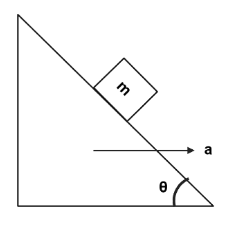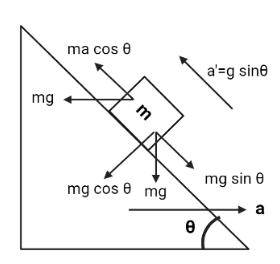Courses
Courses for Kids
Free study material
Free LIVE classes
More# By what acceleration, the wedge should move so that the block moves upwards along the surface of the wedge with an acceleration of $g\sin \theta$.A. $2g\cos \theta$B. $g\sin \theta$C. $2g\tan \theta$D. $g\cos \theta$

Last updated date: 25th Mar 2023
Total views: 207.3k
Views today: 1.84kVerified
207.3k+ views
Hint:To construct a free-body diagram, we draw the point of concern, all forces acting on it, and resolve all force vectors into x– and y–components. A separate free-body diagram is required for each object in the question.$ma\cos \theta - mg\sin \theta = ma' \\ \Rightarrow ma\cos \theta - mg\sin \theta = mg\sin \theta \\ \Rightarrow a\cos \theta = 2g\sin \theta \\ \Rightarrow a = \dfrac{{2g\sin \theta }}{{\cos \theta }} \\ \therefore a = 2g\tan \theta \\$